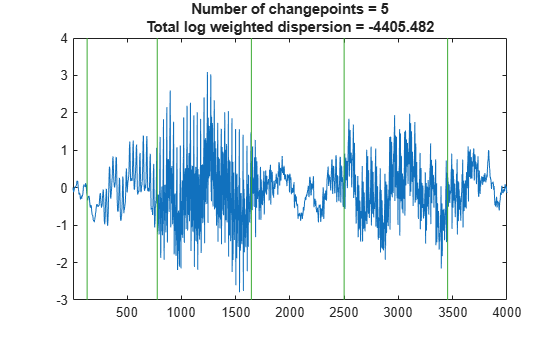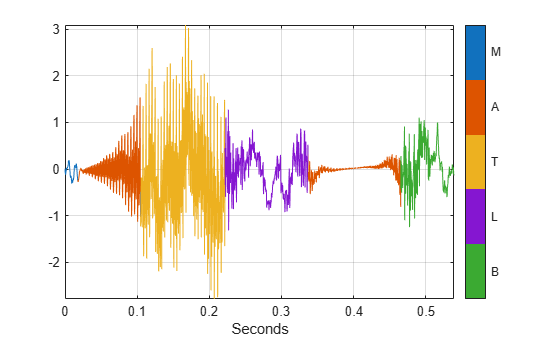Modify and convert signal masks and extract signal regions of interest

## Description

Use `signalMask` to store the locations of regions of interest of a signal together with the label or category values for each region.

Using `signalMask`, you can:

• Represent a signal mask as a table, a categorical sequence, or a matrix of binary sequences.

• Modify regions of interest by extending or shortening their duration, merge same-category regions that are sufficiently close, or remove regions that are not long enough.

• Extract signal regions of interest from a signal vector.

• Plot a signal with color-coded regions of interest.

## Creation

### Syntax

``msk = signalMask(src)``
``msk = signalMask(src,Name,Value)``

### Description

example

````msk = signalMask(src)` creates a signal mask for the input data source `src`. A mask defines the locations of regions of interest of a signal together with the label or category values for each region.```
````msk = signalMask(src,Name,Value)` sets Properties using name-value pairs. You can specify multiple name-value pairs. Enclose each property name in quotes.```

### Input Arguments

expand all

Input data source, specified as a region-of-interest (ROI) table, a categorical vector sequence, or a matrix of binary sequences.

• When `src` is an ROI table, it must contain two variables:

• The first variable is a two-column matrix. Each row of the matrix contains the beginning and end limits of a signal region of interest.

• If `SampleRate` is specified, `signalMask` interprets the limits as time values expressed in seconds.

• If `SampleRate` is not specified, `signalMask` interprets the limits as sample indices. If the matrix elements are not integers, `signalMask` rounds their values to the nearest integer greater than zero.

• The second variable contains the region labels, specified as a categorical array or a string.

• When `src` is a categorical vector sequence, groups of contiguous same-value category elements indicate a signal region of interest labeled with that particular category. Elements that belong to no category (and hence have no label value) should be specified as the missing categorical, displayed as `<undefined>`. For more information, see `categorical`.

• When `src` is a P-column matrix of binary sequences, each column is interpreted as a signal mask with `true` elements marking regions of interest for each of P different categories, labeled with integers from 1 to P. If you prefer, you can specify a list of P category names using the `Categories` property.

Example: `signalMask(table([2 4;6 7],["male" "female"]'))` specifies a signal mask with a three-sample `male` region and a two-sample `female` region.

Example: ```signalMask(categorical(["" "male" "male" "male" "" "female" "female" ""]',["male" "female"]))``` specifies a signal mask with a three-sample `male` region and a two-sample `female` region.

Example: ```signalMask([0 1 1 1 0 0 0 0;0 0 0 0 0 1 1 0]','Categories',["male" "female"])``` specifies a signal mask with a three-sample `male` region and a two-sample `female` region.

Data Types: `single` | `double` | `int8` | `int16` | `int32` | `int64` | `uint8` | `uint16` | `uint32` | `uint64` | `logical` | `char` | `string` | `table` | `categorical`

## Properties

expand all

Type of input source, returned as `"roiTable"`, `"categoricalSequence"`, or `"binarySequences"`. This property is inferred from `src` and cannot be set.

Example: `signalMask(table([2 4;6 7],["male" "female"]'))` has `SourceType` returned as `"roiTable"`.

Example: ```signalMask(categorical(["" "male" "male" "male" "" "female" "female" ""]',["male" "female"]))``` has `SourceType` returned as `"categoricalSequence"`.

Example: ```signalMask([0 1 1 1 0 0 0 0;0 0 0 0 0 1 1 0]','Categories',["male" "female"])``` has `SourceType` returned as `"binarySequences"`.

Data Types: `string`

Sample rate value, specified as a positive numeric scalar. If `src` is specified as an ROI table, `signalMask` assumes that the table contains region limits expressed in seconds. If you omit this property, the object treats all region limits as sample indices.

Data Types: `single` | `double`

Category names, specified as a string vector or a cell array of character vectors. This property can be set only when `src` is a matrix of binary sequences. For all other input `src` types, `signalMask` infers category names directly from `src` and this property is read-only. The vector has a number of elements equal to the number of columns of `src`, and its ith category corresponds to the ith column of `src`. If `src` has P columns and this property is not specified, `signalMask` sets the category names to `["1" "2" ... "P"]`.

Data Types: `string` | `char`

Option to select a subset of categories, specified as a logical value. If this property is set to `false` after creating the mask, then all categories in `Categories` are selected. `SpecifySelectedCategories` can only be used on an existing object and cannot be specified as a name-value pair.

Data Types: `logical`

Indices of selected categories, specified as a vector of integer index values pointing to category elements in `Categories`. Categories not listed in this property are filtered out from the mask input when calling the object functions of `signalMask`. The category indices must be sorted in ascending order. This property applies only when `SpecifySelectedCategories` is `true`. `SelectedCategories` can only be used on an existing object and cannot be specified as a name-value pair.

Example: Given a set of categories `["woman" "girl" "man" "boy"]`, specifying `SelectedCategories` as `[1 2 4]` selects `["woman" "girl" "boy"]` and filters out the rest.

Data Types: `single` | `double` | `int8` | `int16` | `int32` | `int64` | `uint8` | `uint16` | `uint32` | `uint64`

Number of samples to extend regions to the left, specified as a positive integer. The number of extended samples is truncated when the beginning of the sequence is reached. For more information, see Region Limit Modification.

Data Types: `single` | `double` | `int8` | `int16` | `int32` | `int64` | `uint8` | `uint16` | `uint32` | `uint64`

Number of samples to extend regions to the right, specified as a positive integer. For more information, see Region Limit Modification.

Data Types: `single` | `double` | `int8` | `int16` | `int32` | `int64` | `uint8` | `uint16` | `uint32` | `uint64`

Number of samples to shorten regions from the left, specified as a positive integer. `signalMask` removes regions that are shortened by a number of samples equal to or greater than their length. For more information, see Region Limit Modification.

Data Types: `single` | `double` | `int8` | `int16` | `int32` | `int64` | `uint8` | `uint16` | `uint32` | `uint64`

Number of samples to shorten regions from the right, specified as a positive integer. `signalMask` removes regions that are shortened by a number of samples equal to or greater than their length. For more information, see Region Limit Modification.

Data Types: `single` | `double` | `int8` | `int16` | `int32` | `int64` | `uint8` | `uint16` | `uint32` | `uint64`

Distance between regions to be merged, specified as a positive integer. When this property is specified, `signalMask` merges regions of the same category that are separated by the specified number of samples or less. For more information, see Region Limit Modification.

Data Types: `single` | `double` | `int8` | `int16` | `int32` | `int64` | `uint8` | `uint16` | `uint32` | `uint64`

Minimum length of regions to keep, specified as a positive integer. When this property is specified, `signalMask` removes regions shorter than the specified number of samples. For more information, see Region Limit Modification.

Data Types: `single` | `double` | `int8` | `int16` | `int32` | `int64` | `uint8` | `uint16` | `uint32` | `uint64`

## Object Functions

 `binmask` Get matrix of binary sequences mask `catmask` Get categorical sequence mask `extractsigroi` Extract regions of interest based on signal mask `plotsigroi` Plot signal regions based on signal mask `roimask` Get ROI table mask

## Examples

collapse all

Load a speech signal sampled at ${F}_{s}=7418\phantom{\rule{0.2777777777777778em}{0ex}}Hz$. The file contains a recording of a female voice saying the word "MATLAB®."

```load mtlb t = (0:length(mtlb)-1)/Fs;```

Discern the vowels and consonants in the word by finding the points at which the variance of the signal changes significantly. Limit the number of changepoints to five.

`q = findchangepts(mtlb,"Statistic","rms","MaxNumChanges",5);`

Plot the signal and display the changepoints.

```findchangepts(mtlb,"Statistic","rms","MaxNumChanges",5) axis tight```Define regions of interest that correspond to each letter in the word.

`roitable = t([[1;q] [q;length(mtlb)]]);`

Assign region labels and preserve their order.

```x = ["M" "A" "T" "L" "A" "B"]'; y = unique(x,"stable"); c = categorical(x,y);```

Create a signal mask for the regions of interest and corresponding labels. Shorten each region by one sample from the right to avoid contiguity. Display the region-of-interest table mask.

```src = table(roitable,c); msk = signalMask(src,"SampleRate",Fs,"RightShortening",1); roimask(msk)```
```ans=6×2 table roitable c ___________________ _ 0 0.017525 M 0.01766 0.10461 A 0.10475 0.22162 T 0.22176 0.33675 L 0.33688 0.46535 A 0.46549 0.53909 B ```

Introduce gaps in the signal where the letter `"A"` is vocalized.

```m = mtlb; seq = catmask(msk,length(mtlb)); m(seq == "A") = NaN;```

Reconstruct the signal using an autoregressive process. Extract each region of interest from the reconstructed signal.

```p = fillgaps(m); w = extractsigroi(msk,p);```

To play the sound with a pause after each region, uncomment these lines:

```% for k = 1:length(w) % sound(cell2mat(w{k}),Fs) % pause(0.5) % end```

Plot the reconstructed signal and visualize the regions of interest.

```figure plotsigroi(msk,p)```